# Auto and Cross Correlation

June 2, 2021

### Auto-Correlation

The auto-correlation function Rxx(m) for a real-valued sequence x(n) is defined as:

(1)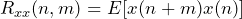If the data sequence x(n) is wide sense stationary, then Rxx(n,n+m) simplifies to:

(2)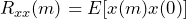The statistical properties do not depend on absolute time n but on time difference m.

Rxx(m) displays how statistically related a data sequence is to an m-sample delayed version of itself. For example, if you look at sample x(3), how strong is its statistical relationship with x(4)? How about x(5) or x(6)?

For many real-world systems, Rxx(m) generally decreases as m increases, meaning the data sequence x becomes less statistically related to itself as the delay increases.

Two properties of Rxx(m) are its center spike and symmetry.

#### Center Spike

Rxx(m) has a spike at the center m=0 location because:

(3)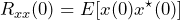(4)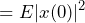(5)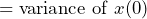#### Symmetry

Rxx(m) is symmetric about the center m=0 location because:

 (6)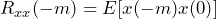by definition of Rxx(m) (7)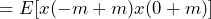by wide sense stationary property (8)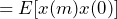by commutative property (9)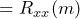by definition of Rxx(m)

### Cross-Correlation

The cross-correlation function Ryx(m) is similar to the autocorrelation Rxx(m), but two sequences x and y are compared rather than solely x.

The cross-correlation function Ryx(m) for a real-valued sequence x(n) is defined as:

(10)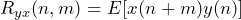If the data sequence x(n) is wide sense stationary, then Ryx(n,n+m) simplifies to:

(11)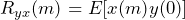The statistical properties do not depend on absolute time n, only on the time difference m.

Ryx(m) displays how statistically related a data sequence x is to an m-sample delayed version of a data sequence y. For example, if you look at sample x(3), how strong is its statistical relationship with y(4)? How about y(6)?